# Box plot visualization with Pandas and Seaborn

Box Plot is the visual representation of the depicting groups of numerical data through their quartiles. Boxplot is also used for detect the outlier in data set. It captures the summary of the data efficiently with a simple box and whiskers and allows us to compare easily across groups. Boxplot summarizes a sample data using 25th, 50th and 75th percentiles. These percentiles are also known as the lower quartile, median and upper quartile.

A box plot consist of 5 things.

• Minimum
• First Quartile or 25%
• Median (Second Quartile) or 50%
• Third Quartile or 75%
• Maximum

Draw the box plot with Pandas:

One way to plot boxplot using pandas dataframe is to use `boxplot()` function that is part of pandas library.

 `# import the required library  ` `import` `numpy as np  ` `import` `pandas as pd  ` `import` `matplotlib.pyplot as plt  ` `%` `matplotlib inline ` ` `  ` `  `# load the dataset ` `df ``=` `pd.read_csv(``"tips.csv"``) ` ` `  `# display 5 rows of dataset ` `df.head()    `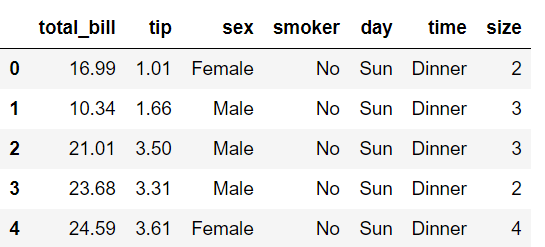Boxplot of `days `with respect `total_bill`.

 `df.boxplot(by ``=``'day'``, column ``=``[``'total_bill'``], grid ``=` `False``) `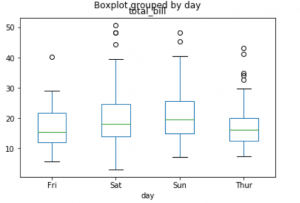Boxplot of `size `with respect `tip`.

 `df.boxplot(by ``=``'size'``, column ``=``[``'tip'``], grid ``=` `False``) `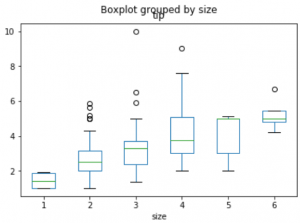Draw the boxplot using seaborn library:

Syntax :
`seaborn.boxplot(x=None, y=None, hue=None, data=None, order=None, hue_order=None, orient=None, color=None, palette=None, saturation=0.75, width=0.8, dodge=True, fliersize=5, linewidth=None, whis=1.5, notch=False, ax=None, **kwargs)`

Parameters:
x = feature of dataset
y = feature of dataset
hue = feature of dataset
data = datafram or full dataset
color = color name

Let’s see how to create the box plot through seaborn library.

 `# load the dataset ` `tips ``=` `sns.load_dataset(``'tips'``) ` ` `  `tips.head() `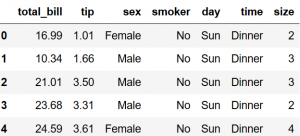Boxplot of `days `with respect `total_bill`.

 `# Draw a vertical boxplot grouped  ` `# by a categorical variable: ` `sns.set_style(``"whitegrid"``) ` ` `  `sns.boxplot(x ``=` `'day'``, y ``=` `'total_bill'``, data ``=` `tips) `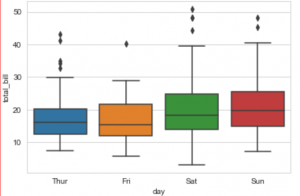Let’s take the first box plot i.e, blue box plot of the figure and understand these statistical things:

• Bottom black horizontal line of blue box plot is minimum value
• First black horizontal line of rectangle shape of blue box plot is First quartile or 25%
• Second black horizontal line of rectangle shape of blue box plot is Second quartile or 50% or median.
• Third black horizontal line of rectangle shape of blue box plot is third quartile or 75%
• Top black horizontal line of rectangle shape of blue box plot is maximum value.
• Small diamond shape of blue box plot is outlier data or erroneous data.

Attention geek! Strengthen your foundations with the Python Programming Foundation Course and learn the basics.

To begin with, your interview preparations Enhance your Data Structures concepts with the Python DS Course.

My Personal Notes arrow_drop_upCheck out this Author's contributed articles.

If you like GeeksforGeeks and would like to contribute, you can also write an article using contribute.geeksforgeeks.org or mail your article to contribute@geeksforgeeks.org. See your article appearing on the GeeksforGeeks main page and help other Geeks.

Please Improve this article if you find anything incorrect by clicking on the "Improve Article" button below.

Article Tags :

3

Please write to us at contribute@geeksforgeeks.org to report any issue with the above content.